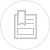# basic priniciples - definitions

Applying a potential differential between two electrodes plunged into an electrolytic bath (solution containing ions) will create a directional electrical field within which ions are put in motion: the cations will move towards the cathode and the anions towards the anode. When a sufficiently strong voltage is applied, the following reactions will take place at the electrolyte-electrode interfaces:

• at the anode: oxidation accompanied by an electron emission
• at the cathode: reduction accompanied by electron capture

## Nernst equation

When an electrode is left in the electrolyte (at zero current), it acquires a voltage E. This voltage, which occurs when the species present are in equilibrium, is called the equilibrium potential and obeys the Nernst equation (see section oxidation-reduction).

where :

E0: the electrode’s equilibrium potential in relation to the standard hydrogen electrode,
E0: The electrode’s equilibrium potential under standard conditions (activity of oxidising and reducing species equal to the unit),
R: ideal gas molar constant,
T: absolute temperature,
n : number of electrons involved in the electro-chemical process,
Aox: activity of the oxidising species,
Ared: activity of the reducing species.

Table 15 provides standard equilibrium potential figures for a few electrochemical couples (at 25°C in relation to the standard hydrogen electrode).

## electrolysis voltage

When an electrolysis cell is operating normally, voltage V obeys a law that has the following form :

where :

E0: electrode equilibrium potential,
s: electrode over voltage,
rI: ohmic drop caused by the electrolyte’s resistivity.

Faraday’s law expresses the relationship that links the amount of electricity passing through an electrolysis cell with the amplitude of the reactions that take place at the electrode-electrolyte interfaces :

where :

P: component mass involved during the reaction (g),
RF.: current efficiency,
M: component’s gramme-molecular weight (g),
I·t: quantity of electricity passing through the cell (C),
n : number of electron-grams exchanged during the reaction,
N: Avogadro’s number = 6.02·1023 for one mole,
e0: electron’s charge = 1.6 10–19 C.